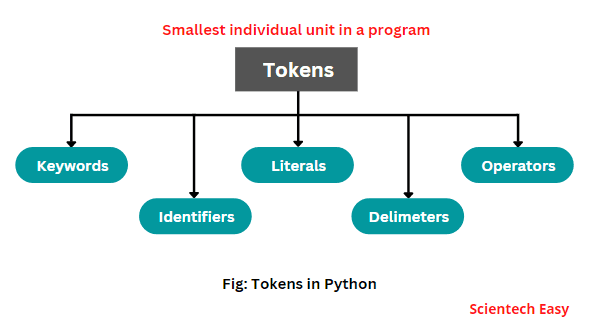# Python Tokens | Character Set Used in Python

Tokens are the various elements in the Python program that are identified by Python interpreter.

A token is the smallest individual unit, or element in the Python program, which is identified by interpreter. They are building blocks of the source code.

Python language supports the different types of tokens that are as follows:

• Keywords (Reserved words) : True, False, None, class, continue, break, if, elif, else, from, or, def, del, import, etc.
• Identifier : User-defined names
• Literals : String, Numeric, Boolean, Collection,
• Delimeters : ( ), { }, [ ], :, ., =, ;, +=, -=, *=, /=, %=, etc.
• Operators : +, -, *, **, /, %, <<, >>, etc.

The following diagram shows you different tokens used in Python.Python interpreter scans written text in the program source code and converts it into tokens during the conversion of source code into machine code.

## How to Identify Tokens in Python Program?

To identify the tokens in the Python program, let us take an example on it. Look at the following simple program code below.

```# Python program to find the subtraction of two numbers.
x = int(input("Enter your first number = "))
y = int(input("Enter your second number = "))
sub = x - y
print("Result = ", sub)
```
```Output:
Enter your first number = 30
Enter your second number = 20
Result =  10
```

Let us consider the first statement, which consists of nine tokens that are as follows:

• x
• =
• int
• (
• input
• (
• “Enter your first number = “
• )
• )

The second statement in the above code contains the following tokens that are as:

• y
• =
• int
• (
• input
• (
• “Enter your second number = “
• )
• )

Tokens in the third statement in the above code is as:

• sub
• =
• x
• y

Note that the Python interpreter ignores # comment symbol and text follows it. Interpreter uses the tokens to detect errors, mainly syntax errors.

When we create a Python program and tokens are not arranged in a particular sequence, then the interpreter produces the error. In the further tutorials, we will discuss the various tokens one by one.

## Character Set Used in Python

The character set included in Python comprises the following characters:

1. Alphabet: It includes the uppercase and lowercase alphabet letters of English, i.e., {A, B, C, D, E, . . . . } and {a, b, c, d, e, f, . . . . }.

2. Digits: It includes the numeric digits, i.e., {0, 1, 2, . . . . 9}.

3. White Spaces: It includes spaces, enters, and tabs.

4. Special Characters: It includes the special symbols, such as {, !, ?, #, <, >, (, ), %, “, &, ^, *, <<, >>, [, ], +, =, /, -, , _, :, ;, }.

In this tutorial, we have elaborated about tokens in Python with example program. Hope that you will have understood the basic points of character set used in Python.